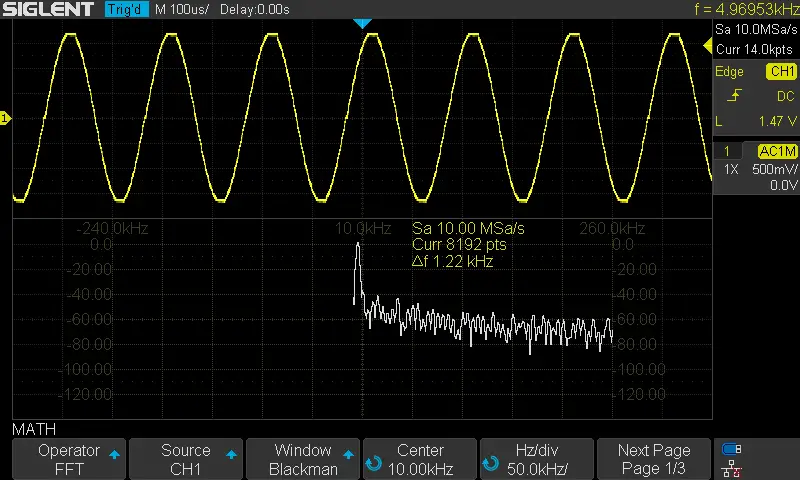﻿ STM32 wave form generator with DAC and DMA, (DAC DMA example) support Sine, Sawtooth, Sinc, etc – 程序员日志

# STM32 wave form generator with DAC and DMA, (DAC DMA example) support Sine, Sawtooth, Sinc, etc

### STM32 DAC WaveForm Generator

• Set up a new project as usual with system clock @ 80MHz
• Set up the DAC1 peripheral to work in normal mode with output buffer enabled
• Use the provided MATLAB script to generate lookup tables to be used for this LAB
• Setup timer2 to be the trigger source for DMA1 unit
• Setup DMA1 to move data from memory to DAC peripheral
• Generate a 1KHz sine waveform. Change the lookup table data to make it sawtooth, triangular, sinc functions

And now, let’s build this system step-by-step

Step1: Open CubeMX & Create New Project

Step2: Choose The Target MCU & Double-Click Its Name

STM32L432KC

Step3: Go To The Clock Configuration

Step4: Set The System Clock To Be 80MHz

Step5: Enable The DAC1 Output In Normal Mode & Buffer Enable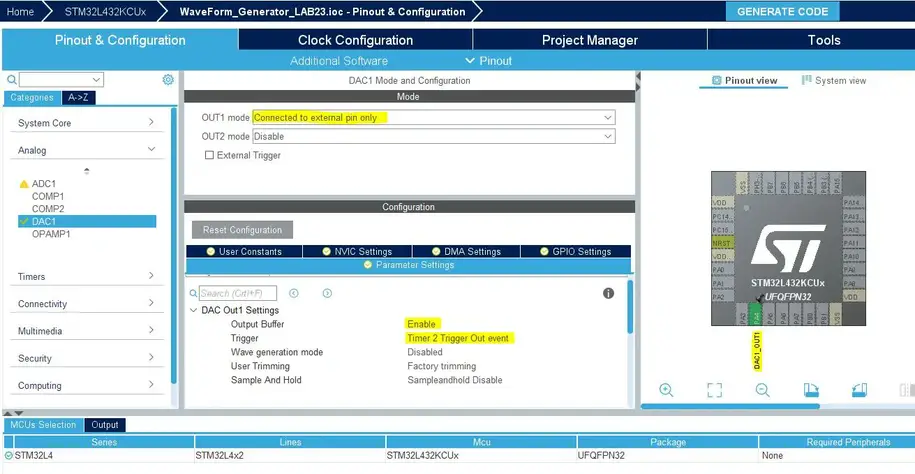Step6: Enable The DAC1 DMA Channel & Configure It As Shown Below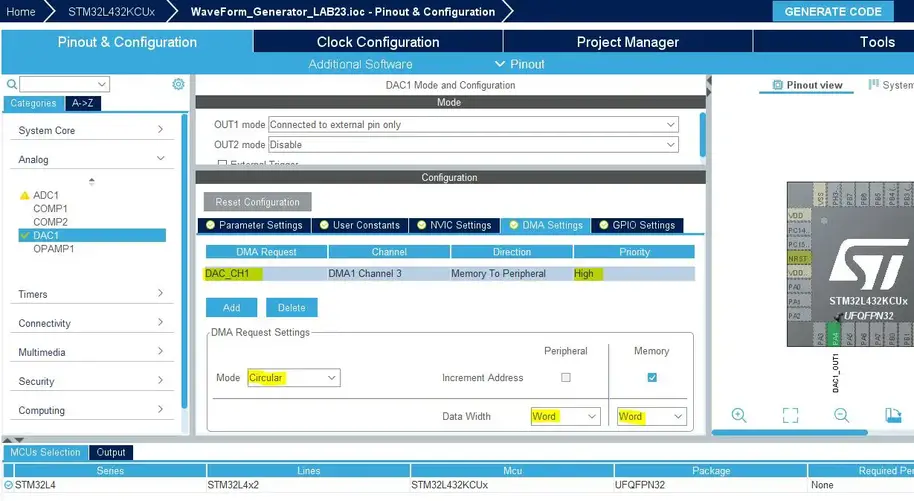Step7: Now, Configure Timer2 Peripheral As Shown

Do you remember the example of the calculation shown earlier in this article? Where we want to generate a 1kHz sine wave with a system clock of 80MHz and lookup table with 128 entries.

The PCS was chosen to be 0, and the ARR was calculated to be 624. That’s what we’re doing here in this step.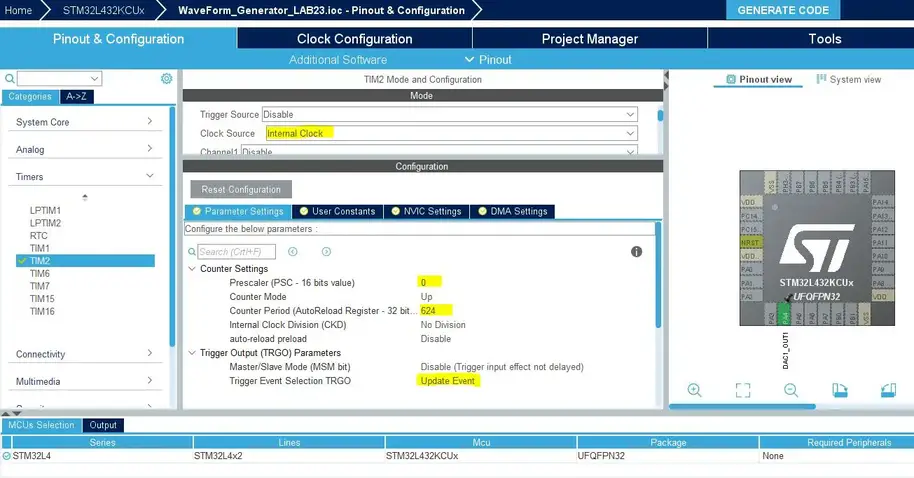Step8: Generate The Initialization Code & Open The Project In Your IDE

Here is The Application Code For This Demo (main.c)

The Result For This LAB Testing On My DSO

Here is the result for the first test (sine wave @ 1KHz)

The FFT shows a THD of nearly 1 or 2% which is not bad. And can be improved by increasing the sample points and adding an offset so that the signal doesn’t swing to the extreme ends.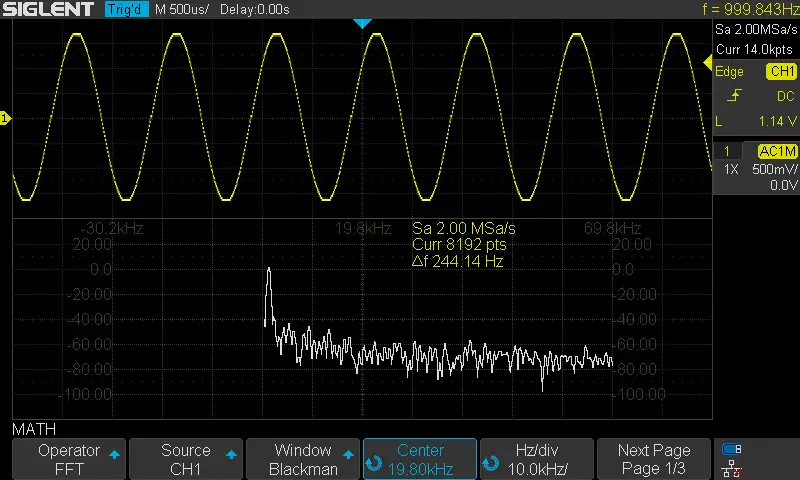Here is the result for the 2nd test (sawtooth wave @ 1KHz)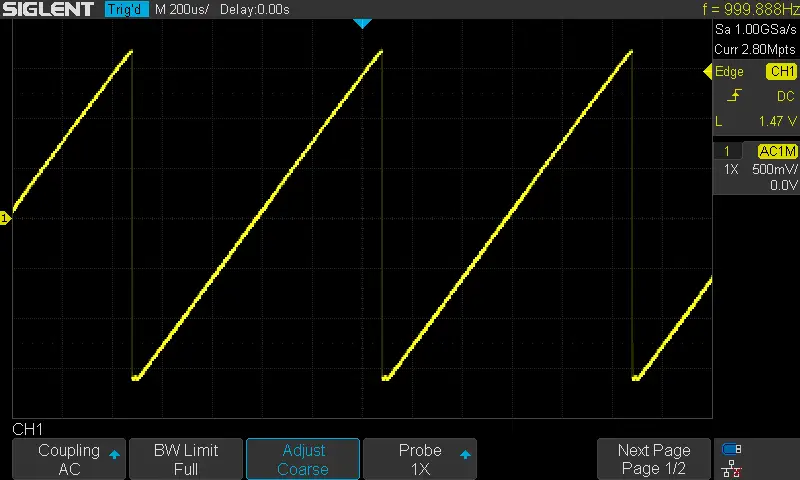Here is the result for the 3rd test (triangular wave @ 1KHz)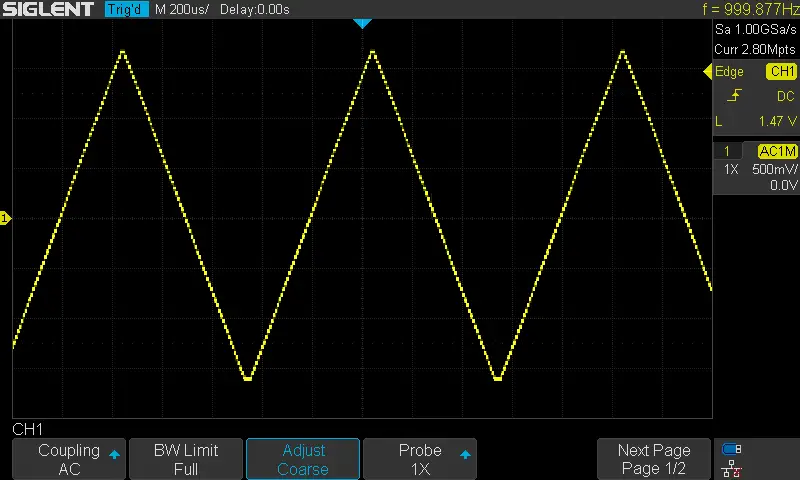Here is the result for the 4th test “The Beautiful Sinc Function”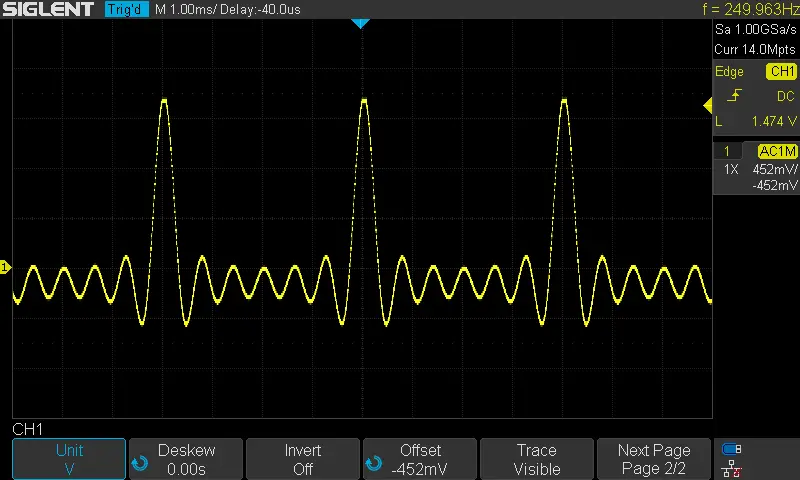Here is the result for the 5th test (sine wave @ 5KHz)

By writing 125 to the Timer2 ARR, we’d expect the sine output signal’s frequency to increase to 5KHz. And that’s exactly what happens.